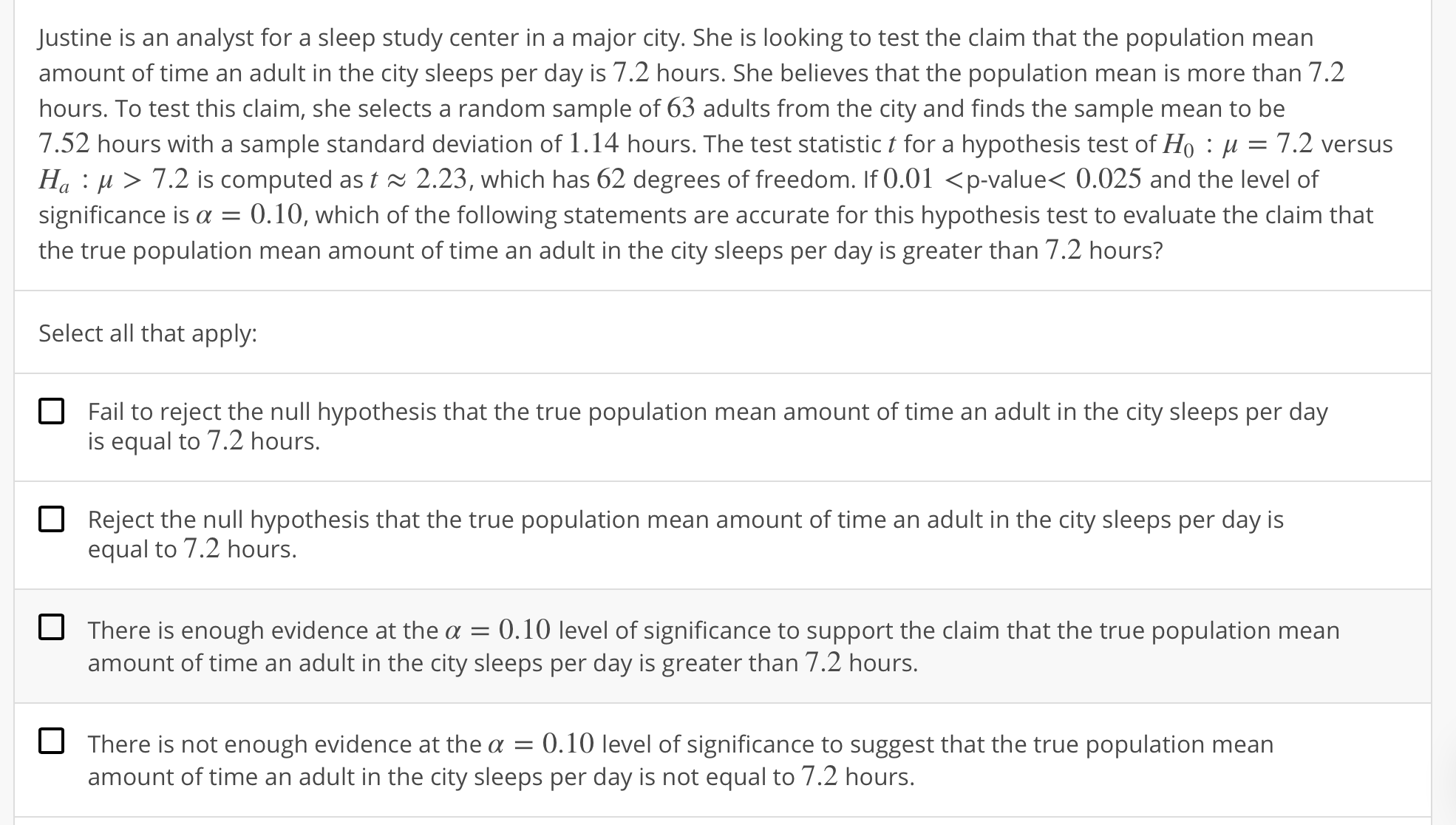Justine is an analyst for a sleep study center in a major city. She is looking to test the claim that the population meanamount of time an adult in the city sleeps per day is 7.2 hours. She believes that the population mean is more than 7.2hours. To test this claim, she selects a random sample of 63 adults from the city and finds the sample mean to be7.52 hours with a sample standard deviation of 1 . 14 hours. The test statistic t for a hypothesis test of Ho : μ = 7.2 versusHa : μ 〉 7.2 is computed as t 2.23, which has 62 degrees of freedom. If 0.01 〈p-value< 0.025 and the level ofsignificance is α = 0.10, which of the following statements are accurate for this hypothesis test to evaluate the claim thatthe true population mean amount of time an adult in the city sleeps per day is greater than 7.2 hours?Select all that apply:Fail to reject the null hypothesis that the true population mean amount of time an adult in the city sleeps per dayis equal to 7.2 hours.Reject the null hypothesis that the true population mean amount of time an adult in the city sleeps per day isequal to 7.2 hours.OThere is enough evidence at the α-0. 10 level of significance to support the claim that the true population meanamount of time an adult in the city sleeps per day is greater than 7.2 hours.There is not enough evidence at the a 0.10 level of significance to suggest that the true population meanamount of time an adult in the city sleeps per day is not equal to 7.2 hours.

Questionhelp_outlineImage TranscriptioncloseJustine is an analyst for a sleep study center in a major city. She is looking to test the claim that the population mean amount of time an adult in the city sleeps per day is 7.2 hours. She believes that the population mean is more than 7.2 hours. To test this claim, she selects a random sample of 63 adults from the city and finds the sample mean to be 7.52 hours with a sample standard deviation of 1 . 14 hours. The test statistic t for a hypothesis test of Ho : μ = 7.2 versus Ha : μ 〉 7.2 is computed as t 2.23, which has 62 degrees of freedom. If 0.01 〈p-value< 0.025 and the level of significance is α = 0.10, which of the following statements are accurate for this hypothesis test to evaluate the claim that the true population mean amount of time an adult in the city sleeps per day is greater than 7.2 hours? Select all that apply: Fail to reject the null hypothesis that the true population mean amount of time an adult in the city sleeps per day is equal to 7.2 hours. Reject the null hypothesis that the true population mean amount of time an adult in the city sleeps per day is equal to 7.2 hours. O There is enough evidence at the α-0. 10 level of significance to support the claim that the true population mean amount of time an adult in the city sleeps per day is greater than 7.2 hours. There is not enough evidence at the a 0.10 level of significance to suggest that the true population mean amount of time an adult in the city sleeps per day is not equal to 7.2 hours. fullscreen
Step 1

Decision based on p-value:

At level of significance α, if the p-value ≤ α, then reject the null hypothesis, H0. Otherwise fail to reject H0.

If H0 is rejected, then it can be interpreted that “there is enough evidence to suggest that H0 is false, that is, to support the claim made by the alternate hypothesis, Ha at level of significance α”.

Step 2

Interpretation:

Here, the level of significance is α = 0.10 and 0.01 < p-value < 0.025. Evidently, the p-value is less than α. Thus, the claim made by the null hypothesis is rejected. As a result, the 2nd and 3rd interpretation is correct.

Now, the 1st interpretation contradicts the 2nd interpretation. This means that the 1st interpretation must be incorrect when...

Want to see the full answer?

See Solution

Want to see this answer and more?

Our solutions are written by experts, many with advanced degrees, and available 24/7

See Solution
Tagged in

Hypothesis Testing